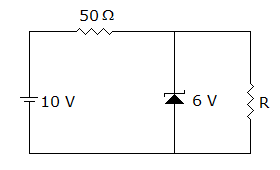# Electronics and Communication Engineering - Electronic Devices and Circuits - Discussion

Discussion Forum : Electronic Devices and Circuits - Section 6 (Q.No. 37)
37.
The 6 V zener diode shown in figure has zero zener resistance and a knee current of 5 mA. The minimum value of R, so that the voltage across it does not fall below 6 V is1.2 kΩ
80 Ω
50 W
0
Explanation:
No answer description is available. Let's discuss.
Discussion:
5 comments Page 1 of 1.

Matha said:   4 years ago

Markkevin94 said:   4 years ago
Nice one. Thanks for the explanation.

MAY JOY SIDAYON said:   4 years ago
Source current, Is = (10-6)/50 = 80 mA.

Knee current = 5 mA.
So, load current = 80 - 5 = 75 mA.

Hence, the minimum load resistance = 6/75 = 80Ω

Japh said:   7 years ago
Thank you, @Vishal Deep.

Vishal deep said:   9 years ago
Source current, Is = (10-6)/50 = 80 mA.

Knee current = 5 mA.
So load current = 80-5 = 75 mA.

Hence minimum load resistance = 6/75 = 80Ω